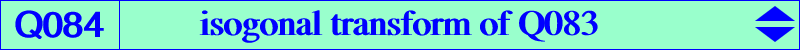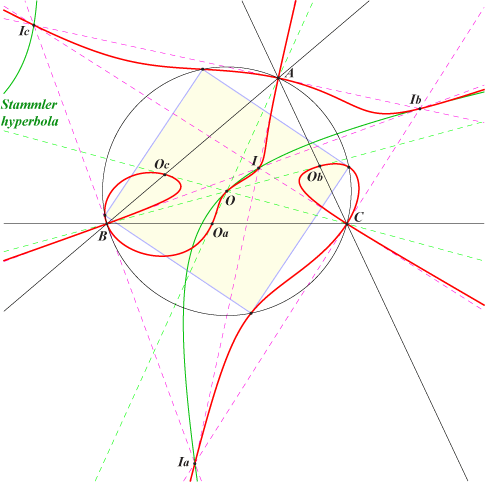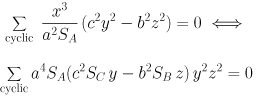X(1), X(3), X(2635)* excenters extraversions of X(2635)* vertices of the cevian triangle of OQ084 is the isogonal transform of the stelloidal quartic Q083. It follows that Q084 is a quintic meeting the circumcircle at A, B, C and at the vertices of a square. A, B, C are nodes with tangents the bisectors of ABC. K373 is the locus of points whose polar conic in Q084 is a rectangular hyperbola. In other words, the cubic is the Laplacian of the quintic.